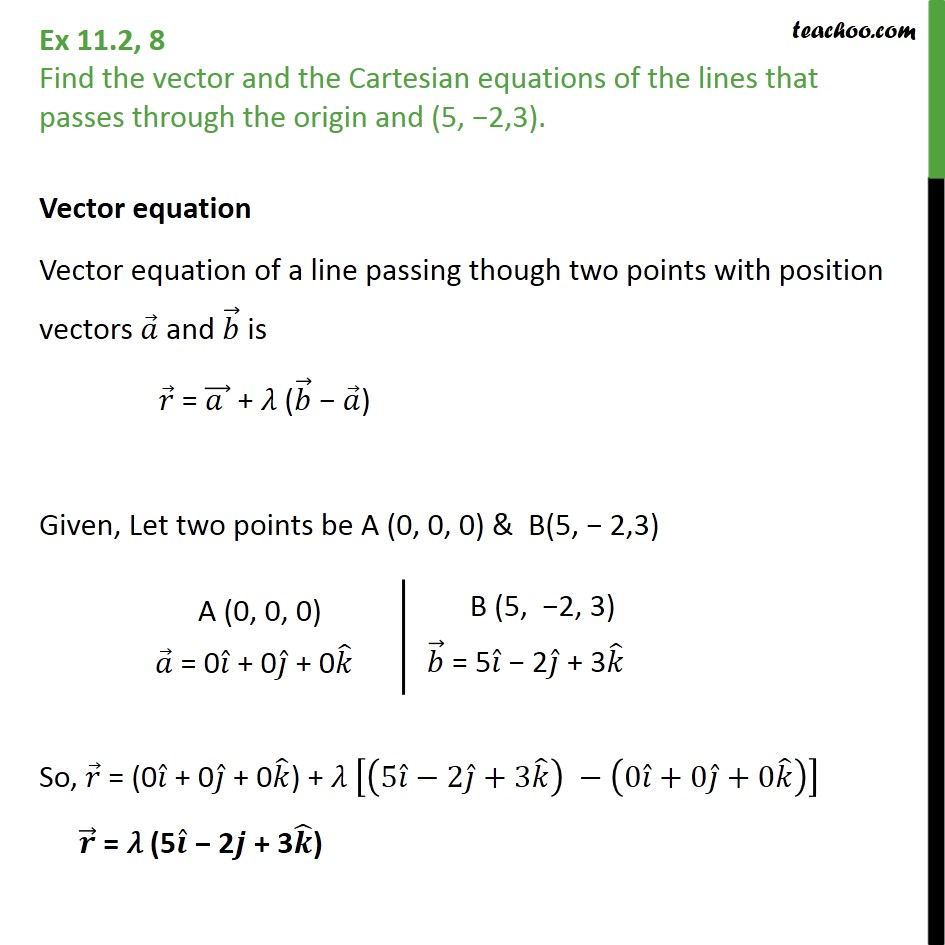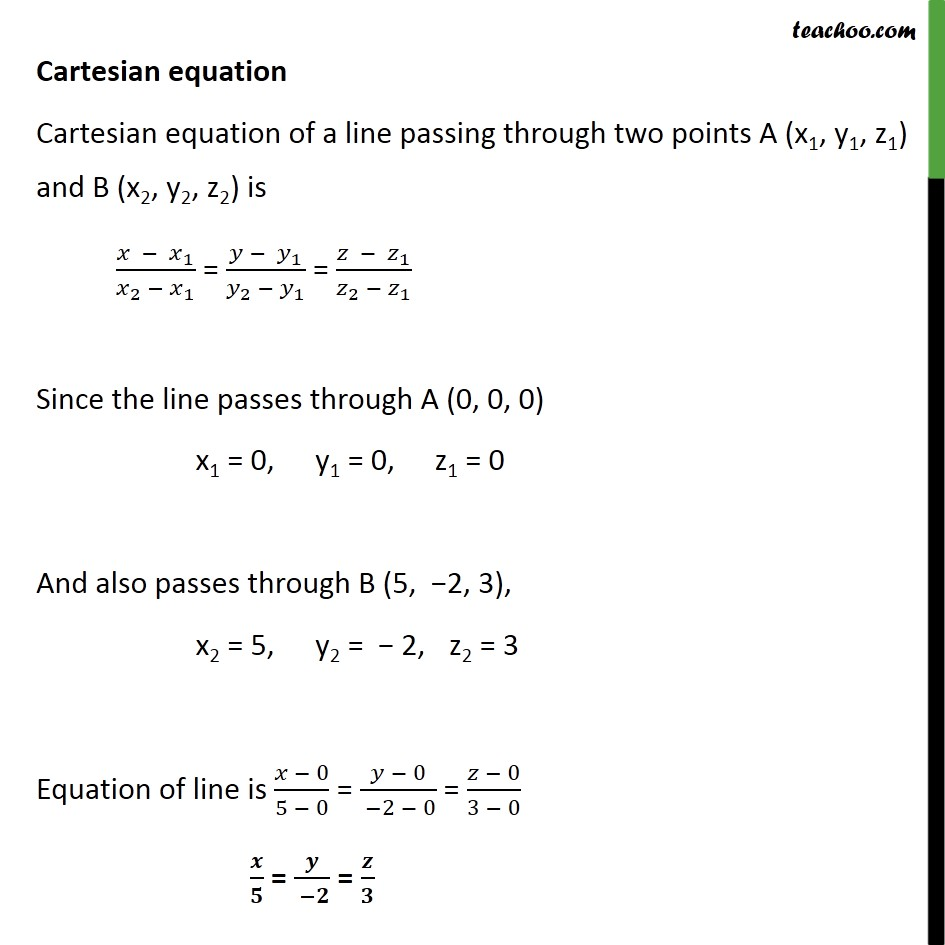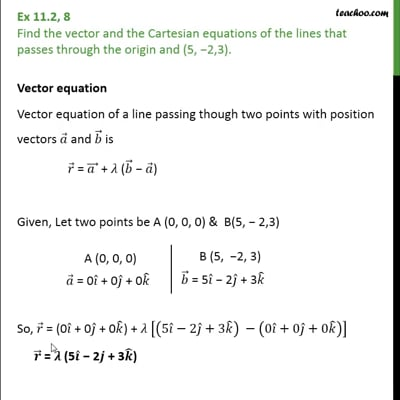Ex 11.2

Chapter 11 Class 12 Three Dimensional Geometry
Serial order wiseThis video is only available for Teachoo black users

Solve all your doubts with Teachoo Black (new monthly pack available now!)

### Transcript

Ex 11.2, 8 Find the vector and the Cartesian equations of the lines that passes through the origin and (5, 2,3). Vector equation Vector equation of a line passing though two points with position vectors and is = + ( ) Given, Let two points be A (0, 0, 0) & B(5, 2,3) So, = (0 + 0 + 0 ) + 5 2 +3 0 +0 +0 = (5 2 + 3 ) Cartesian equation Cartesian equation of a line passing through two points A (x1, y1, z1) and B (x2, y2, z2) is 1 2 1 = 1 2 1 = 1 2 1 Since the line passes through A (0, 0, 0) x1 = 0, y1 = 0, z1 = 0 And also passes through B (5, 2, 3), x2 = 5, y2 = 2, z2 = 3 Equation of line is 0 5 0 = 0 2 0 = 0 3 0 = =